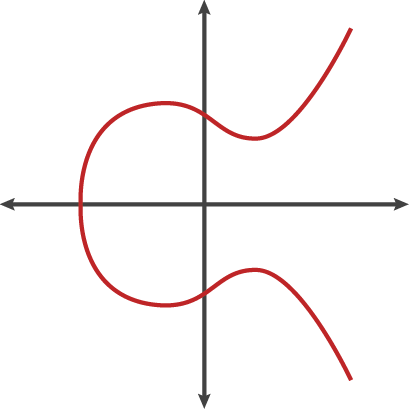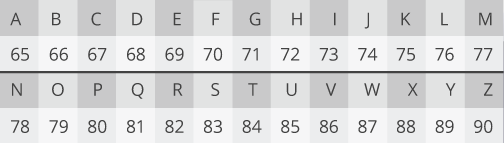# A (relatively easy to understand) primer on elliptic curve cryptography Cloudflar

Readers are reminded that elliptic curve cryptography is a set of algorithms for encrypting and decrypting data and exchanging cryptographic keys.

Dual_EC_DRBG, the cryptographic standard suspected of containing a backdoor engineered by the National Security Agency, is a function that uses elliptic curve mathematics to generate a series of random-looking numbers from a seed. This primer comes two months after internationally recognized cryptographers called on peers around the world to adopt ECC to avert a possible “cryptopocalypse.”

By NICK SULLIVANNICK SULLIVAN

Elliptic curve cryptography (ECC) is one of the most powerful but least understood types of cryptography in wide use today.

An increasing number of websites make extensive use of ECC to secure everything from customers’ HTTPS connections to how they pass data between data centers. Fundamentally, it’s important for end users to understand the technology behind any security system in order to trust it. To that end, we looked around to find a good, relatively easy-to-understand primer on ECC in order to share with our users. Finding none, we decided to write one ourselves. That is what follows.

Be warned: this is a complicated subject, and it’s not possible to boil it down to a pithy blog post. In other words, settle in for a bit of an epic because there’s a lot to cover.

If you just want the gist, here’s the TL;DR version: ECC is the next generation of public key cryptography, and based on currently understood mathematics, it provides a significantly more secure foundation than first-generation public key cryptography systems like RSA. If you’re worried about ensuring the highest level of security while maintaining performance, ECC makes sense to adopt. If you’re interested in the details, read on.

### The dawn of public key cryptographyElliptic curve cryptography (ECC)

The history of cryptography can be split into two eras: the classical era and the modern era. The turning point between the two occurred in 1977, when both the RSA algorithm and the Diffie-Hellman key exchange algorithm were introduced. These new algorithms were revolutionary because they represented the first viable cryptographic schemes where security was based on the theory of numbers; it was the first to enable secure communication between two parties without a shared secret. Cryptography went from being about securely transporting secret codebooks around the world to being able to have provably secure communication between any two parties without worrying about someone listening in on the key exchange.

### Whitfield Diffie and Martin HellmanWhitfield Diffie and Martin Hellman.

Modern cryptography is founded on the idea that the key that you use to encrypt your data can be made public while the key that is used to decrypt your data can be kept private. As such, these systems are known as public key cryptographic systems. The first, and still most widely used of these systems, is known as RSA—named after the initials of the three men who first publicly described the algorithm: Ron Rivest, Adi Shamir, and Leonard Adleman.

What you need for a public key cryptographic system to work is a set of algorithms that is easy to process in one direction but difficult to undo. In the case of RSA, the easy algorithm multiplies two prime numbers. If multiplication is the easy algorithm, its difficult pair algorithm is factoring the product of the multiplication into its two component primes. Algorithms that have this characteristic—easy in one direction, hard the other—are known as trapdoor functions. Finding a good trapdoor function is critical to making a secure public key cryptographic system. Simplistically, the bigger the spread between the difficulty of going one direction in a trapdoor function and going the other, the more secure a cryptographic system based on it will be.

### A toy RSA algorithm

The RSA algorithm is the most popular and best understood public key cryptography system. Its security relies on the fact that factoring is slow and multiplication is fast. What follows is a quick walk-through of what a small RSA system looks like and how it works.

In general, a public key encryption system has two components, a public key and a private key. Encryption works by taking a message and applying a mathematical operation to it to get a random-looking number. Decryption takes the random looking number and applies a different operation to get back to the original number. Encryption with the public key can only be undone by decrypting with the private key.

Computers don’t do well with arbitrarily large numbers. We can make sure that the numbers we are dealing with do not get too large by choosing a maximum number and only dealing with numbers less than the maximum. We can treat the numbers like the numbers on an analog clock. Any calculation that results in a number larger than the maximum gets wrapped around to a number in the valid range.

In RSA, this maximum value (call it max) is obtained by multiplying two random prime numbers. The public and private keys are two specially chosen numbers that are greater than zero and less than the maximum value (call them pub and priv). To encrypt a number, you multiply it by itself pub times, making sure to wrap around when you hit the maximum. To decrypt a message, you multiply it by itself priv times, and you get back to the original number. It sounds surprising, but it actually works. This property was a big breakthrough when it was discovered.

To create an RSA key pair, first randomly pick the two prime numbers to obtain the maximum (max). Then pick a number to be the public key pub. As long as you know the two prime numbers, you can compute a corresponding private key priv from this public key. This is how factoring relates to breaking RSA—factoring the maximum number into its component primes allows you to compute someone’s private key from the public key and decrypt their private messages.

Let’s make this more concrete with an example. Take the prime numbers 13 and 7. Their product gives us our maximum value of 91. Let’s take our public encryption key to be the number 5. Then using the fact that we know 7 and 13 are the factors of 91 and applying an algorithm called the Extended Euclidean Algorithm, we get that the private key is the number 29.

These parameters (max: 91, pub: 5, priv: 29) define a fully functional RSA system. You can take a number and multiply it by itself 5 times to encrypt it, then take that number and multiply it by itself 29 times and you get the original number back.

### Let’s use these values to encrypt the message “CLOUD”.

In order to represent a message mathematically, we have to turn the letters into numbers. A common representation of the Latin alphabet is UTF-8. Each character corresponds to a number.Under this encoding, CLOUD is 67, 76, 79, 85, 68. Each of these digits is smaller than our maximum of 91, so we can encrypt them individually. Let’s start with the first letter.

We have to multiply it by itself five times to get the encrypted value.

67×67 = 4489 = 30 *

*Since 4489 is larger than max, we have to wrap it around. We do that by dividing by 91 and taking the remainder.

4489 = 91×49 + 30

30×67 = 2010 = 8

8×67 = 536 = 81

81×67 = 5427 = 58

This means the encrypted version of 67 (or C) is 58.

Repeating the process for each of the letters, we get that the encrypted message CLOUD becomes:

58, 20, 53, 50, 87

To decrypt this scrambled message, we take each number and multiply it by itself 29 times:

58×58 = 3364 = 88 (Remember, we wrap around when the number is greater than max.)

88×58 = 5104 = 8

9×58 = 522 = 67

Voila, we’re back to 67. This works with the rest of the digits, resulting in the original message.

The takeaway is that you can take a number, multiply it by itself a number of times to get a random-looking number, and then multiply that number by itself a secret number of times to get back to the original number.

A (relatively easy to understand) primer on elliptic curve cryptography
Everything you wanted to know about the next generation of public key crypto. – By NICK SULLIVAN – 10/24/2013

Nick Sullivan

Author Nick Sullivan worked for six years at Apple on many of its most important cryptography efforts before recently joining CloudFlare, where he is a systems engineer. He has a degree in mathematics from the University of Waterloo and a Masters in computer science with a concentration in cryptography from the University of Calgary. This post was originally written for the CloudFlare blog and has been lightly edited to appear on Ars.

Readers are reminded that elliptic curve cryptography is a set of algorithms for encrypting and decrypting data and exchanging cryptographic keys. Dual_EC_DRBG, the cryptographic standard suspected of containing a backdoor engineered by the National Security Agency, is a function that uses elliptic curve mathematics to generate a series of random-looking numbers from a seed. This primer comes two months after internationally recognized cryptographers called on peers around the world to adopt ECC to avert a possible “cryptopocalypse.

Fonte: ARSTECHNICA

Digital Certificates Explained – Basics – Animated

Novo marco da ICP-Brasil: emitidas Cadeias V6 e V7 em curvas elípticas

Elliptic Curve Cryptography. A Importância das Curvas Elípticas

AC-Raiz e cadeias V6 e V7. Parece rotina. Só que não!

Padrões e requisitos de segurança da ICP-Brasil estarão presentes nas urnas eletrônicas em 2020

Blockchain Synthesis – By ITI Brazil